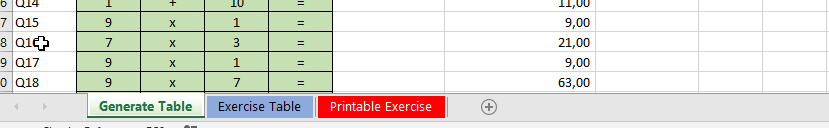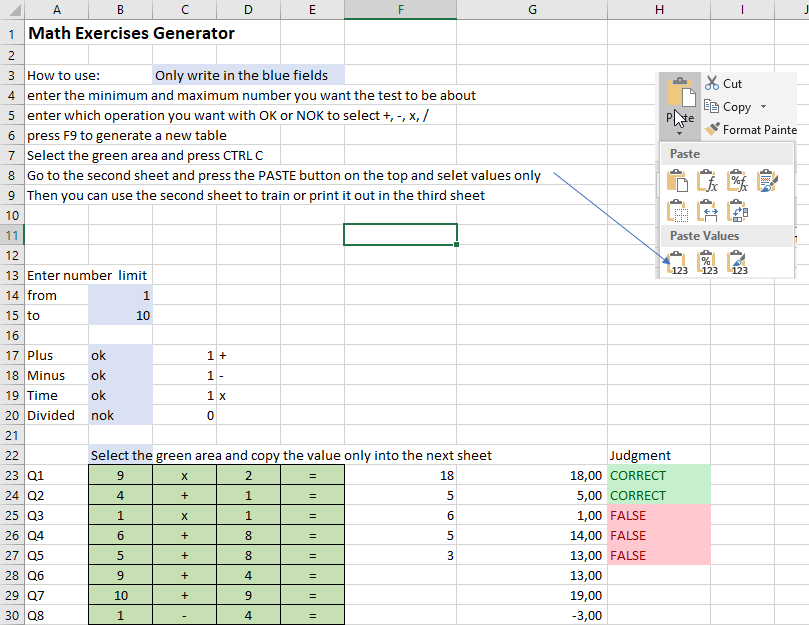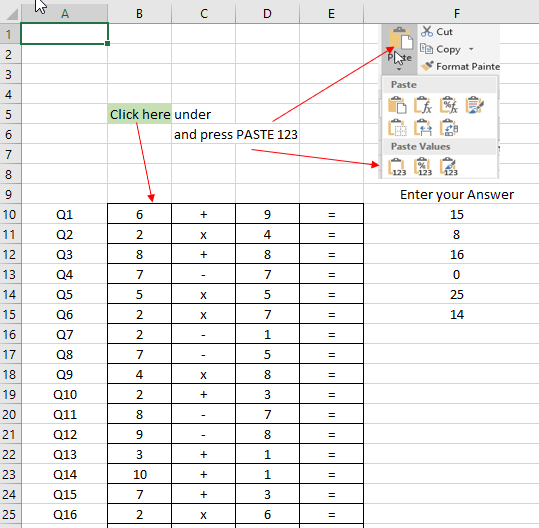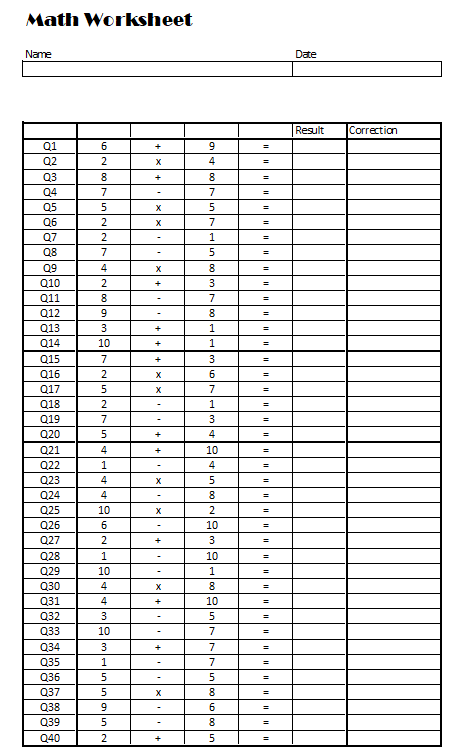## Math worksheet to learn basic operations

Math is an important topic to learn and teaching it well takes time. We provide you here with some help with an excel sheet that can generate worksheet for training your kid at home or your pupils at school. It can also be a math quiz or math test for your students.

This math calculation worksheet is made of three parts.Part one is the Generator. It create the calculation table in Excel.

Part 2 is the Exercise Table and part 3 is the printablemath worksheet.You first select in the math calculation worksheet the boundaries. Like numbers between 1 and 10 or numbers between 10 and 100 or 10 and 20, etc… this depends on the current abilities of the students.

Then select the operation: if your students only know basic operation like addition, then just select the “plus” by entering OK in the plus blue cell and leave the other one empty. If you want to use also subtraction or multiplication or division then add OK next these.

This will create per default 40 operations. To use it, copy the green area into the next sheet by only copying the text as explained in the sheet or on this page “how to convert a formula to text”.To finally print it out, use the 3rd sheet that will let you print out the full exercise.We hope you like our calculation worksheet for first, second graders and for you if you want to train bigger numbers head calculation.